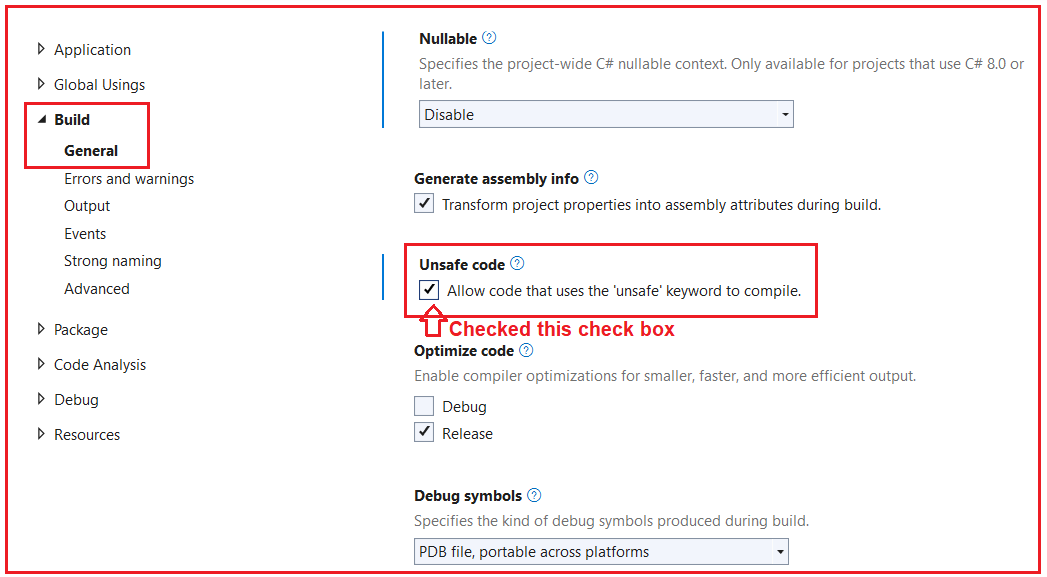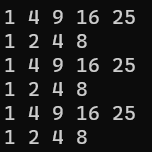# Stackalloc in in C#

## Stackalloc in C# with Examples

In this article, I am going to discuss Stackalloc in C# 8 with Examples. Please read our previous article where we discussed Unmanaged Constructed Types in C# 8 with Examples. In this article, I will show you how to use Stackalloc before C# 7.2, how to use it in C# 7.2 and 7.3, and what enhancements have been done in C# 8 with Examples.

##### What is stackalloc in C#?

The stackalloc operator in C# creates a block of memory on the stack and returns a pointer to the start of that memory address. Stack-allocated memory is automatically destroyed when the scope it was created in is exited. You cannot explicitly free the memory allocated with stackalloc.

Note: The stackalloc operator allocates memory in an unsafe context (so it should be used with caution). It is similar to the alloc function of our traditional C language. The stackalloc operator implements a form of malloc that frees the memory when the calling function returns.

##### Example to Understand stackalloc Before C# 7.2 :

Let us see an example to understand stackalloc in C# before C# 7.2. For a better understanding, please have a look at the following example. This example uses an unsafe method. So, in order to compile it, you must enable unsafe code. In this example, the stackalloc operator allocates 40 bytes of memory on the stack. Here, you can see, we have written the code using the unsafe block.

```using System;
namespace Csharp8Features
{
using System;

class Program
{
static void Main()
{
//Before C# 7.2
unsafe
{
//Allocate Some Memory on the stack using stackalloc
//Int = 4 Bytes, so it will allocate 40 (10*4) Bytes of Memory in Stack
int* ptr = stackalloc int;

for (int i = 0; i < 10; i++)
{
ptr[i] = i + 1;
}

for (int i = 0; i < 10; i++)
{
Console.Write(\$"{ptr[i]} ");
}
}

}
}
}
```

To compile this program, we need to enable unsafe mode using the project properties window. So, first, open the Project Properties window and then go to the build tab and checked the unsafe check box as shown in the below image and save it.Now, with the above changes in place, you can compile and run the unsafe.

##### Example to Understand stackalloc in C# 7.2:

Let us see an example to understand stackalloc in C# 7.2. From C# 7.2, you can assign the result of a stackalloc expression to either System.Span<T> or System.ReadOnlySpan<T> without using an unsafe context. For a better understanding, please have a look at the following example. This is the same example as the previous one, but in this example, we are removing the unsafe block.

```using System;
namespace Csharp8Features
{
using System;

class Program
{
static void Main()
{
//C# 7.2

//Allocate Some Memory on the stack using stackalloc
//Int = 4 Bytes, so it will allocate 40 (10*4) Bytes of Memory in Stack
//Using Span<int>, so unsafe block is not required
Span<int> ptr = stackalloc int;

for (int i = 0; i < 10; i++)
{
ptr[i] = i + 1;
}

for (int i = 0; i < 10; i++)
{
Console.Write(\$"{ptr[i]} ");
}

}
}
}
```
##### Example to Understand stackalloc in C# 7.3:

Let us see an example to understand stackalloc in C# 7.3. From C# 7.3, stackalloc can be used for initializing arrays. For a better understanding, please have a look at the following example. In the below example, I am showing how to initialize an array with and without using stackalloc operator.

```using System;
namespace Csharp8Features
{
using System;

class Program
{
static void Main()
{
//C# 7.3

//Initialzing Array without stackalloc
var arr1 = new int { 1, 4, 9, 16, 25 };
for (int i = 0; i < 5; i++)
{
Console.Write(\$"{arr1[i]} ");
}
Console.WriteLine();
var arr2 = new int[] { 1, 2, 4, 8 };
for (int i = 0; i < 4; i++)
{
Console.Write(\$"{arr2[i]} ");
}
Console.WriteLine();
//Initialzing Array with stackalloc
unsafe
{
int* pArr1 = stackalloc int { 1, 4, 9, 16, 25 };
for (int i = 0; i < 5; i++)
{
Console.Write(\$"{pArr1[i]} ");
}
Console.WriteLine();
int* pArr2 = stackalloc int[] { 1, 2, 4, 8 };
for (int i = 0; i < 4; i++)
{
Console.Write(\$"{pArr2[i]} ");
}
}
Console.WriteLine();
//Initialzing Array with stackalloc and Span<T>
//Here, unsafe block is not required
Span<int> ptr1 = stackalloc int { 1, 4, 9, 16, 25 };
for (int i = 0; i < 5; i++)
{
Console.Write(\$"{ptr1[i]} ");
}
Console.WriteLine();
Span<int> ptr2 = stackalloc int[] { 1, 2, 4, 8 };
for (int i = 0; i < 4; i++)
{
Console.Write(\$"{ptr2[i]} ");
}

}
}
}
```
###### Output:Note: With C# 7.2, we started using Span<T>, ReadOnlySpan<T>, and Memory<T> because they are ref-struct instances that are guaranteed to be allocated on the stack, and therefore won’t affect the garbage collector.

##### Example to Understand stackalloc in C# 8:

Starting with C# 8.0, if the result of a stackalloc expression is of the System.Span<T> or System.ReadOnlySpan<T> type, you can use the stackalloc expression in other expressions. For a better understanding, please have a look at the below example.

```using System;
using System.Dynamic;
using System.Reflection;

namespace Csharp8Features
{
public class StackMemoryAllocation
{
public static void Main()
{
//Storing the result of stackalloc in Span<int>
Span<int> numbers = stackalloc int[] { 10, 20, 30, 40, 50, 60, 70, 80, 80, 100 };

//Now we can use stackalloc expression i.e. numbers in other expressions
//IndexOfAny: Searches for the first index of any of the specified values.
var index = numbers.IndexOfAny(stackalloc[] {11, 40, 60, 100 });

Console.WriteLine(index); // output: 3
}
}
}
```

Output: 3

##### Another Example to Understand nested stackalloc in C# 8:

Please have a look at the below example for a better understanding.

```using System;
using System.Drawing;
using System.Reflection;

namespace Csharp8Features
{
public class StackMemoryAllocation

{
public static void Main()
{
//Storing the result of stackalloc in Span<int> so that we can resue it in another expression
Span<int> set = stackalloc int { 1, 2, 3, 4, 5, 6 };

//Reusing stackalloc expression
//Forms a slice out of the current span starting at a specified index for a specified length.
Span<int> subSet = set.Slice(3, 2);

foreach (var n in subSet)
{
Console.WriteLine(n); // Output: 4 5
}
}
}
}
```
##### When to use stackalloc in C#?

The stackalloc should only be used for performance optimizations (either for computation or interop). This is due to the following facts:

1. The garbage collector is not required as the memory is allocated on the stack rather than the heap. The memory is released as soon as the variable goes out of scope.
2. It is faster to allocate memory on the stack rather than the heap.

In the next article, I am going to discuss the C# 9 Features with Examples. Here, in this article, I try to explain Stackalloc in C# 8 with Examples. I hope you enjoy this Stackalloc in C# with Examples article.